# Test: General Organic Chemistry- 1

## 20 Questions MCQ Test Organic Chemistry | Test: General Organic Chemistry- 1

Description
This mock test of Test: General Organic Chemistry- 1 for Chemistry helps you for every Chemistry entrance exam. This contains 20 Multiple Choice Questions for Chemistry Test: General Organic Chemistry- 1 (mcq) to study with solutions a complete question bank. The solved questions answers in this Test: General Organic Chemistry- 1 quiz give you a good mix of easy questions and tough questions. Chemistry students definitely take this Test: General Organic Chemistry- 1 exercise for a better result in the exam. You can find other Test: General Organic Chemistry- 1 extra questions, long questions & short questions for Chemistry on EduRev as well by searching above.
QUESTION: 1

### Which of the following is not a resonance structure of the others?

Solution:

Option 'D' is correct because there in no conjugation of negative charge and without conjugation resonance is not possible.

QUESTION: 2

### The number of sigma and pi-bonds in 1-butene 3-yne are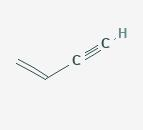Solution: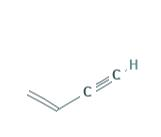Single bond contains only a sigma bond, One double bond contains a sigma and a pi bond, whereas a triple bond contains a sigma bond and 2 pi bonds.
So, CH2 = CH – C ≡ CH
No of σ bonds = 2 + 1 + 1 + 1 +1 + 1 = 7;
No of π bonds = 1 + 2 = 3.

QUESTION: 3

### Which of the following molecules has all the effects : inductive, mesomeric and Baker Nathan effect ?

Solution:

In CH3​CH=CHC(=O)CH3​, presence of CH3​CH= group shows no bond resonance (Baker nathan effect), presence of alternate π bond and electron negative O shows mesomeric effect and CH3​  positive inductive effect and C=O group shows negative inductive effect.

QUESTION: 4

The correct order of electron cloud in benzene ring for the following compounds is: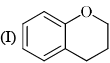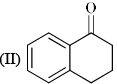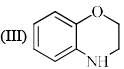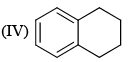Solution:

In case of 3 lone pair of O and N both delocalise into benzene ring so it has maximum e- density.

In case of 1 lone pair of O delocalise into benzene ring so it has second highest e- density.

In case of 2, Due to the presence of carbonyl oxygen atom ,it pulls the electron density from the benzene ring through delocalisation.

In 4, there is no such effect.

QUESTION: 5

Most stable resonating structure of the given carbocation is: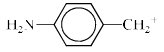Solution:

Because in option 'C' all the atoms have their octets fulfilled which is not in the case with the other options.

QUESTION: 6

Which of the following resonance structures contributes the most to the resonance hybrid?

Solution:

Most stable structure contributes more in the resonance hybrid. Here we can see that option 'D' has 3 double bonds in it it which  means that it is the most stable among all. So it will be the most contributing structure in resonance hybrid than others.

QUESTION: 7

Stability order of the following resonating structure will be: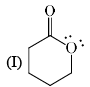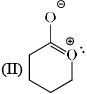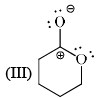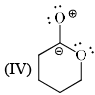Solution:

-1st is uncharged so it is the most stable among the following options.
-2nd one is charged but have more no of covalent bonds than 3rd and 4th so it is more stable than 3rd and 4th.
-Between 3rd and 4th follow the electronegativity rulle which means more electronegative atom bears negative charge. In 4th the positive charge on oxygen atom is against the electronegative prinicple . So it will like to have negative charge rather than positive chargee. So 3rd is more stable tha 4th.
Therefore the order will be 1>2>3>4

QUESTION: 8

Hyperconjugation is best described as:

Solution:

Hyperconjugation is the stabilising interaction that results from the interaction of the electrons in a σ-bond (usually C-H or C-C) with an adjacent empty or partially filled p-orbital or a π-orbital to give an extended molecular orbital that increases the stability of the system.

QUESTION: 9

The correct stability order for the following species is: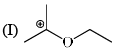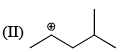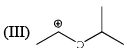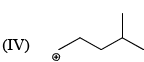Solution:

Carbocation is resonance stabilized when it is adjacent to an oxygen atom.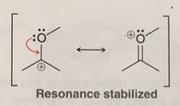Therefore, 1 and 3 are more stable than 2 and 4 and among 1 and 3 1 is more stable due to +I effect of -CH3 also.

Stability of hydrocarbons increase in the order of primary,secondary and then tertiary. Therefore 2 is more stable than 4.

QUESTION: 10

Increasing order of pKa values (pKa = –log Ka) of H2O, CH3OH and C6H5OH is:

Solution:

Explanation :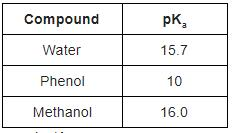pKa values are -logKa.

Ka is the dissociation constant of the acid

Smaller the Ka value, weaker is the acid.

Therefore, pka values will go on decreasing from strong acid to weak acid.

QUESTION: 11

Identify order of per ring resonance energies of each of the following: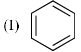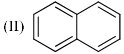Solution:

As the number of the rings increases,the resonance energy per ring decreases. So option 1 is correct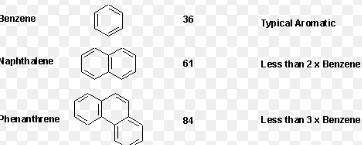QUESTION: 12

Identify the correct C-O bond length order: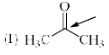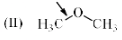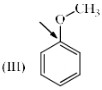Solution:

Explanation : The triple bonds are the strongest and hence the shortest. Then comes double bonds which are of intermediate strength between the triple and single bonds. And finally the single bonds are weaker than the other two. This way, Triple bonds are the shortest.

Option I) is the shortest.

In III the lone pair over the Oxygen atom is involve in conjugation so it has partial double bond character, means bond length is lesser than single bond but greater than double bond. so bond length of III is greater than II.

QUESTION: 13

Which of the following systems are resonance contributors of the radical shown below?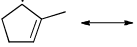Solution:
QUESTION: 14

Which of the following statements about the resonance structures is false?

Solution:

Hydrogens must have two electrons and elements in the second row cannot have more than 8 electrons. If so, the resonance structure is not valid. Always look at the placement of arrows to make sure they agree.

QUESTION: 15

Which comparison is not correct as indicated?

Solution: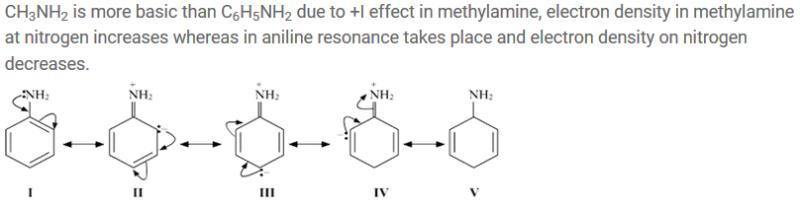QUESTION: 16

The acidity order for the following compound follows the order: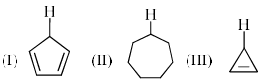Solution:

Explanation : Acidity of a compound depends upon the stability of the conjugate base.Decreasing order of the stability is  aromatic, homoaromatic, non aromatic, anti aromatic.

Ist conjugate base is aromatic

2nd conjugate base is non aromatic

3rd conjugate base is anti aromatic

QUESTION: 17

In the following compounds the order of acid strength is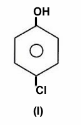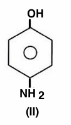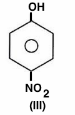Solution:

Nitro and chlorine act as electro withdrawing group which increases the acidic character in compound where as NH2 acts as electron donating which decreases the acidic character. Further nitro is strong withdrawing group so first priority is give to para nitro phenol ,afterwards para chloro phenol. So correct answer is (a)

QUESTION: 18

The acidity of protons H in each of the following is: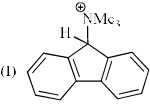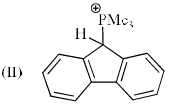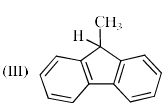Solution:

In case of molecule 1 nitrogen captures the negative charge after protonation i.e. delocalization of the -ve charge in aromatic polycyclic ring will be less but in case of molecule 2, P have less electronegativity and hence delocalization of -ve charge can occur more easily within the aromatic rings. and in case of 3 the +I effect of CH3 disturbs the stability of -ve charge form after protonation.

QUESTION: 19

Identify the correct acidic strength order in the following compounds?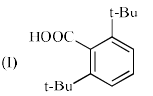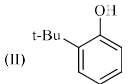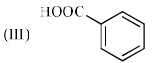Solution:

In 1, the ortho effect is highly effective and hence is most acidic among II AND III . In option II, the tertiary butoxy group increases the electrohillic nature of benezene ring and hence the negative charge formed on phenoxide ion after the removal of proton gets more dispersed in the ring and thus the anion gets stable and becomes more acidic then III.( benzoic acid).
Thus Correct option is A

QUESTION: 20

Identify the correct order of boiling points of the following compounds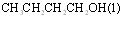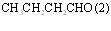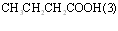Solution:

Carboxylic acids, similar to alcohols, can form hydrogen bonds with each other as well as van der Waals dispersion forces and dipole-dipole interactions. So Max. boiling point. is of carboxylic acid.

The boiling point of aldehydes and ketones are lower than alcohols due to absence of intermolecula hydogen bonding. Alcohol Contains an OH Group Allowing For Hydrogen Bonding Which Is Stronger Than Other Intermolecular Forces.